## Summer Break is Near

 /* styles */ This Week at Northside will also be on break, but we'll be sure to let you know about any important items that come up. Best wishes to you and your families for a safe and happy summer break.
 table div table+table+table+table div table{width:100%;padding:0}table div table+table+table+table div table img{width:96.23%;padding:0;float:none}table div table+table+table+table div table td{width:100%;padding:0 1.88% 18px}/* styles */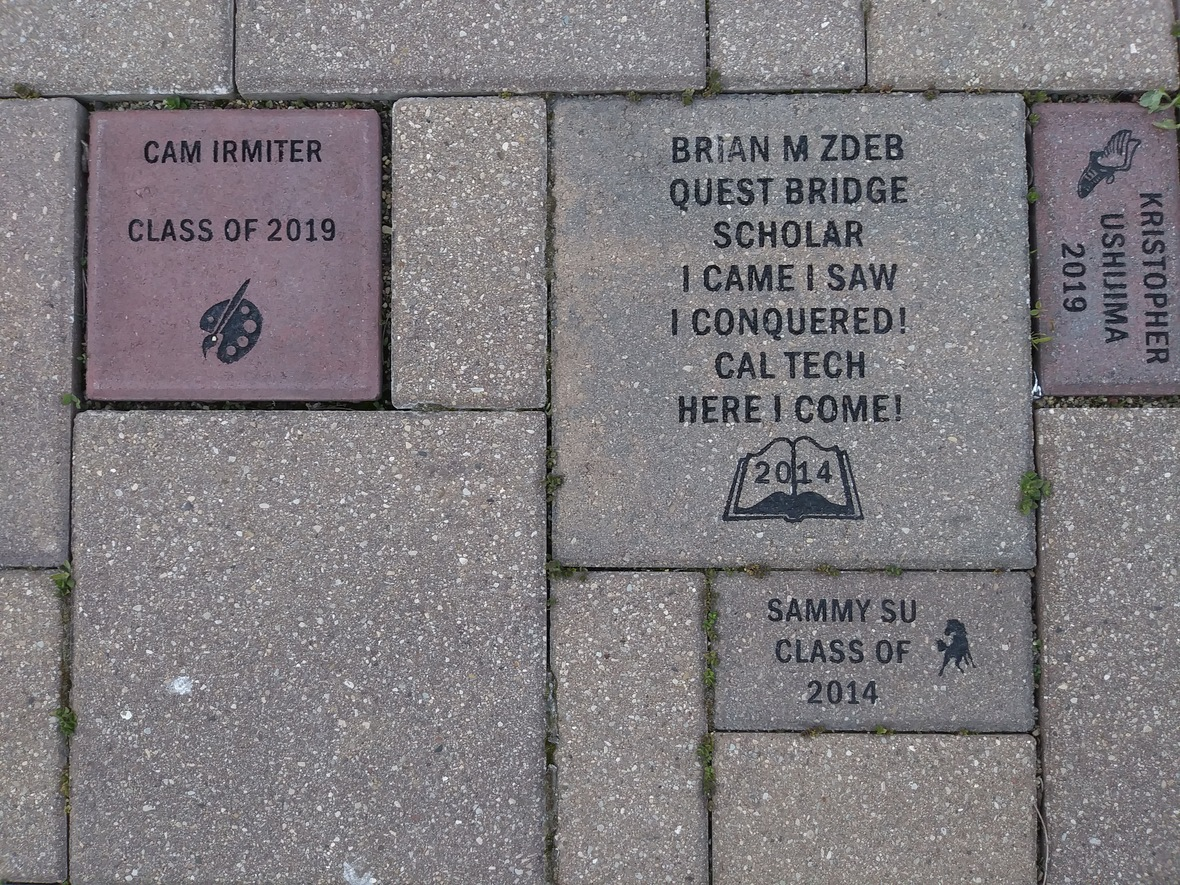Your brick, engraved with a personal message, will become a permanent part of the Northside College Prep Celestial Garden Walkway.

Your brick may be purchased in three sizes:

▪ 4" x 8" = \$50
▪ 8" x 8" = \$100
▪ 12" x 12" = \$150
 ▪ 4" x 8" = \$50
 ▪ 8" x 8" = \$100
 ▪ 12" x 12" = \$150

 table div table+table+table+table+table+table div table{width:100%;padding:0}table div table+table+table+table+table+table div table img{width:96.23%;padding:0;float:none}table div table+table+table+table+table+table div table td{width:100%;padding:0 1.88% 18px}/* styles */## We Need You During the 2020-2021 School Year# Become a FON Board Member

Friends of Northside (FON) is a parent organization that works to support the Northside community through fundraising and volunteerism. All parents and guardians are automatically members of FON. Board positions are available for those who would like to take a more active role.

FON's fundraising seeks to raise money with the goals of replacing lost funding from CPS, supporting the school’s mission, and maintaining and enhancing the learning experience for all NCP students. FON efforts include the Annual Appeal, Spring Benefit, and Cubby Walk Walk-a-thon, among others.

FON also provides volunteers and hospitality to the NCP community. It plans social/educational programs, recruits volunteers for various NCP events such as the Open House, distributes this weekly email communication, and maintains the student directory.

FON is seeking volunteers to:
* Chair next year's Spring Benefit to be held March 2021
* Oversee Fundraising Benefits
* Coordinate Fundraising Activities
* Lead First Friday Parent Discussions
* Assist in writing the This Week at Northside e-blast.
* and many more positions

No experience required.

To see all the volunteer opportunities listed for 2020-2021 please click HERE to view them on the Friends of Northside website.

If you're interested in joining the board or helping with any of positions listed, please email Matt Schechter, president of FON, at matthew.d.schechter@gmail.com.

 table div table+table+table+table+table+table+table+table+table div table{width:100%;padding:0}table div table+table+table+table+table+table+table+table+table div table img{width:96.23%;padding:0;float:none}table div table+table+table+table+table+table+table+table+table div table td{width:100%;padding:0 1.88% 18px}/* styles */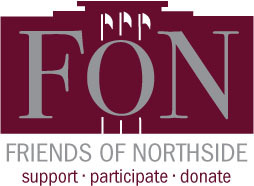# Friends of Northside Zoom Meeting

Tuesday, June 16 at 7:00 pm

All are welcome to attend our virtual Friends of Northside Zoom meeting. Details are below:

▪ Topic: NCP FON June Zoom Meeting
▪ Time: Jun 16, 2020 07:00 PM Central Time (US and Canada)
▪ Join Zoom Meeting: https://us04web.zoom.us/j/75287380901?pwd=UUtUcVZoTWN1TlAvUDd0R0xTUUU4QT09
▪ Meeting ID: 752 8738 0901
 ▪ Topic: NCP FON June Zoom Meeting
 ▪ Time: Jun 16, 2020 07:00 PM Central Time (US and Canada)
 ▪ Join Zoom Meeting: https://us04web.zoom.us/j/75287380901?pwd=UUtUcVZoTWN1TlAvUDd0R0xTUUU4QT09
 ▪ Meeting ID: 752 8738 0901
 table div table+table+table+table+table+table+table+table+table+table+table div table{width:100%;padding:0}table div table+table+table+table+table+table+table+table+table+table+table div table img{width:96.23%;padding:0;float:none}table div table+table+table+table+table+table+table+table+table+table+table div table td{width:100%;padding:0 1.88% 18px}/* styles */# Parent Questions - For Parents Only

Where to Go with Questions

Do you have a general question that you would like addressed in the weekly update? You can submit your question HERE.

Do you have a specific question that will require a follow up?

Take advantage of our virtual office hours and email us at northsideprep@cps.edu or call 773-534-3954 (this voicemail is checked daily, Monday through Friday).

Do you have a question about a class or your student's level of engagement during remote learning?

 table div table+table+table+table+table+table+table+table+table+table+table+table+table+table div table{width:100%;padding:0}table div table+table+table+table+table+table+table+table+table+table+table+table+table+table div table img{width:96.23%;padding:0;float:none}table div table+table+table+table+table+table+table+table+table+table+table+table+table+table div table td{width:100%;padding:0 1.88% 18px}/* styles *//* styles */ Follow Northside on Instagram: https://www.instagram.com/northsidecollegeprep/ Follow NCPSeniors2020 on Instagram: https://www.instagram.com/ncpseniors2020/
 table div table+table+table+table+table+table+table+table+table+table+table+table+table+table+table+table div table{width:100%;padding:0}table div table+table+table+table+table+table+table+table+table+table+table+table+table+table+table+table div table img{width:96.23%;padding:0;float:none}table div table+table+table+table+table+table+table+table+table+table+table+table+table+table+table+table div table td{width:100%;padding:0 1.88% 18px}/* styles */# Student Item Retrieval & Drop-Off

Sophomores - June 15, 16
Freshman - June 17, 18

Click HERE to view the plan for pick up student personal items and drop off any instructional materials (including books, novels, art supplies, and musical instruments). Please read through all of the information carefully as there are strict guidelines that have been put into place by Chicago Public Schools.

Students who are unable to retrieve their items or drop off materials during their assigned slot will be unable to do so outside of that time and will have to wait until either the fall or when CPS allows individuals to enter the building.

 table div table+table+table+table+table+table+table+table+table+table+table+table+table+table+table+table+table+table div table{width:100%;padding:0}table div table+table+table+table+table+table+table+table+table+table+table+table+table+table+table+table+table+table div table img{width:96.23%;padding:0;float:none}table div table+table+table+table+table+table+table+table+table+table+table+table+table+table+table+table+table+table div table td{width:100%;padding:0 1.88% 18px}/* styles */## Yearbooks are Still for Sale!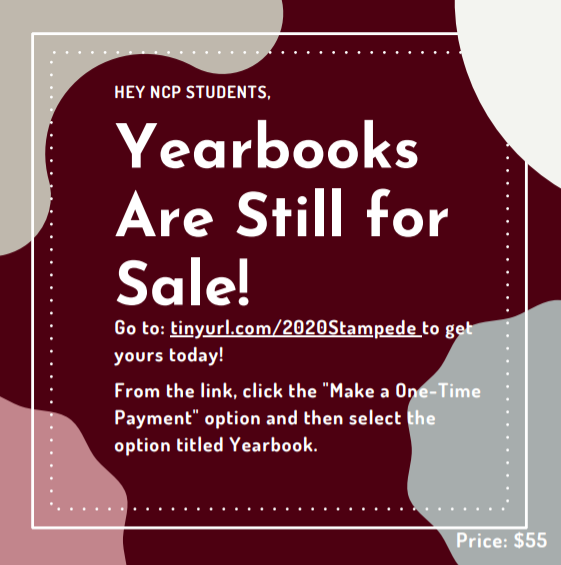/* styles */ Due to COVID-19, Lifetouch has had government-mandated shut-downs in some of their production facilities and social distancing protocols in others that have reduced their capacity for printing yearbooks. Be assured that everyone who ordered a yearbook will receive one. However, the delivery of your yearbook has been delayed. Once we have received the yearbooks, a distribution plan will be shared based upon CPS protocols and guidance.
 table div table+table+table+table+table+table+table+table+table+table+table+table+table+table+table+table+table+table+table+table+table+table div table{width:100%;padding:0}table div table+table+table+table+table+table+table+table+table+table+table+table+table+table+table+table+table+table+table+table+table+table div table img{width:96.23%;padding:0;float:none}table div table+table+table+table+table+table+table+table+table+table+table+table+table+table+table+table+table+table+table+table+table+table div table td{width:100%;padding:0 1.88% 18px}/* styles */## Congratulations to the Class of 2020!/* styles */ On Monday, June 8, the class of 2020 graduated from Northside College Prep. Members of the class will attend some of the nation’s top colleges and universities this fall, and many of them have been awarded a significant amount of scholarships. Congratulations! Go Mustangs!
 table div table+table+table+table+table+table+table+table+table+table+table+table+table+table+table+table+table+table+table+table+table+table+table+table+table+table div table{width:100%;padding:0}table div table+table+table+table+table+table+table+table+table+table+table+table+table+table+table+table+table+table+table+table+table+table+table+table+table+table div table img{width:96.23%;padding:0;float:none}table div table+table+table+table+table+table+table+table+table+table+table+table+table+table+table+table+table+table+table+table+table+table+table+table+table+table div table td{width:100%;padding:0 1.88% 18px}/* styles */# College Conferences

Hello, Class of 2021 Parents,

As we prepare to conclude the school year, the counseling department would like to remind parents and students to schedule their College Conferences. Prior to scheduling your College Conference or obtaining a letter of recommendation, all rising seniors are required to submit their Class of 2021 Self Assessment. The Self Assessment plays an integral role in your school counselor’s ability to prepare for your College Conference and write your letter of recommendation.

To complete your Class of 2021 Self Assessment or Parent Recommendation, follow the steps below:

▪ In the top right-hand corner click on “About Me”
▪ “My Surveys”
▪ “Surveys Not Started”
▪ "Class of 2021 Self Assessment" or "Parent Recommendation"
 ▪ In the top right-hand corner click on “About Me”
 ▪ “My Surveys”
 ▪ “Surveys Not Started”
 ▪ "Class of 2021 Self Assessment" or "Parent Recommendation"

If you are unable to log-in to your Naviance account, e-mail Ms. Fernandez at safernandez@cps.edu.

 table div table+table+table+table+table+table+table+table+table+table+table+table+table+table+table+table+table+table+table+table+table+table+table+table+table+table+table+table+table div table{width:100%;padding:0}table div table+table+table+table+table+table+table+table+table+table+table+table+table+table+table+table+table+table+table+table+table+table+table+table+table+table+table+table+table div table img{width:96.23%;padding:0;float:none}table div table+table+table+table+table+table+table+table+table+table+table+table+table+table+table+table+table+table+table+table+table+table+table+table+table+table+table+table+table div table td{width:100%;padding:0 1.88% 18px}/* styles *//* styles */ FON is working with NCP faculty and staff to coordinate an effort to support the businesses of Northside families. Please use THIS FORM to submit information about a Northside family business. Then we will share the list with the NCP community.
 table div table+table+table+table+table+table+table+table+table+table+table+table+table+table+table+table+table+table+table+table+table+table+table+table+table+table+table+table+table+table+table+table div table{width:100%;padding:0}table div table+table+table+table+table+table+table+table+table+table+table+table+table+table+table+table+table+table+table+table+table+table+table+table+table+table+table+table+table+table+table+table div table img{width:96.23%;padding:0;float:none}table div table+table+table+table+table+table+table+table+table+table+table+table+table+table+table+table+table+table+table+table+table+table+table+table+table+table+table+table+table+table+table+table div table td{width:100%;padding:0 1.88% 18px}/* styles */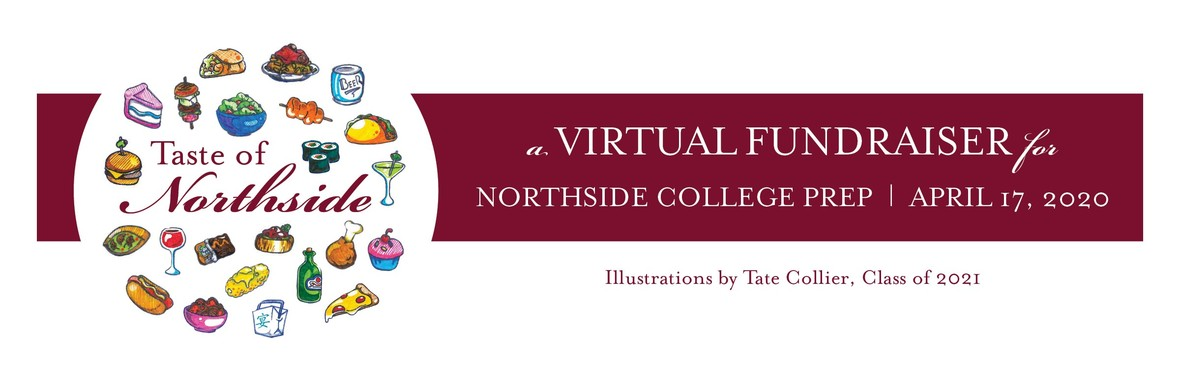# Please Continue to Support the Paddle Raise to Build a New Turf Field

This year’s Paddle Raise is dedicated to raising funds for a much-needed, new turf athletic field. Every year, more gym classes and home athletic games must be cancelled because our Northside field is in such poor condition. Heavy use over the years has rendered it virtually unusable. A new turf field will allow our students, and future Northsiders, to play and exercise outside safely throughout the year. Let's give all of them a home-field advantage. Please demonstrate your strong support for this long-overdue improvement to the Northside campus by making a monetary contribution to the 2020 Paddle Raise.

Thanks to the overwhelming support of our NCP community, we are able to set a new Paddle Raise goal of \$40,000!

Participate in the Paddle Raise for NCP's new turf field. Our Northside video club, the Mustang Reel, created THIS VIDEO to inspire your generosity. Let's all PONY UP, and then click HERE to see the impact of your contribution.

Thank you to those of you who have contacted Kristen Werries Collier to coordinate picking up the silent auction items that you won. If you have not done so, please email her at kristenwerriescollier@gmail.com.

Pickups are taking place in a safe, social-distancing manner from the front porch of her Wicker Park home. For those of you who do not want to venture out, Kristen will continue to hold your items and future arrangements will be made.

# Parent Parties

Thank you to everyone who signed up to participate in a NCP Parent Hosted Party. We are working with the party hosts to reschedule the parties as soon as the stay-at-home order has been lifted. When the time comes that we can gather together again, we will re-open the parties for purchase and let everyone know the details. Thank you for your patience!

 /* styles */ To pay any remaining open balance, please go to Northside.GiveSmart.com. Thank you for your support!
 /* styles */ Questions? Email Debra Swan, Event Chair, at debra@theswans.net.
 table div table+table+table+table+table+table+table+table+table+table+table+table+table+table+table+table+table+table+table+table+table+table+table+table+table+table+table+table+table+table+table+table+table+table+table+table+table+table+table+table+table div table{width:100%;padding:0}table div table+table+table+table+table+table+table+table+table+table+table+table+table+table+table+table+table+table+table+table+table+table+table+table+table+table+table+table+table+table+table+table+table+table+table+table+table+table+table+table+table div table img{width:96.23%;padding:0;float:none}table div table+table+table+table+table+table+table+table+table+table+table+table+table+table+table+table+table+table+table+table+table+table+table+table+table+table+table+table+table+table+table+table+table+table+table+table+table+table+table+table+table div table td{width:100%;padding:0 1.88% 18px}/* styles */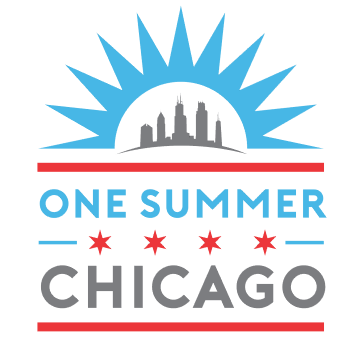# One Summer Chicago Application Is Open!

## Summer Jobs Available for Students - Apply Online

One Summer Chicago brings together government institutions, community-based organizations, and companies to offer over 30,000 employment and internship opportunities
to youth and young adults ages 14 to 24.

Students can apply HERE.

 table div table+table+table+table+table+table+table+table+table+table+table+table+table+table+table+table+table+table+table+table+table+table+table+table+table+table+table+table+table+table+table+table+table+table+table+table+table+table+table+table+table+table+table+table div table{width:100%;padding:0}table div table+table+table+table+table+table+table+table+table+table+table+table+table+table+table+table+table+table+table+table+table+table+table+table+table+table+table+table+table+table+table+table+table+table+table+table+table+table+table+table+table+table+table+table div table img{width:96.23%;padding:0;float:none}table div table+table+table+table+table+table+table+table+table+table+table+table+table+table+table+table+table+table+table+table+table+table+table+table+table+table+table+table+table+table+table+table+table+table+table+table+table+table+table+table+table+table+table+table div table td{width:100%;padding:0 1.88% 18px}/* styles */table div table+table+table+table+table+table+table+table+table+table+table+table+table+table+table+table+table+table+table+table+table+table+table+table+table+table+table+table+table+table+table+table+table+table+table+table+table+table+table+table+table+table+table+table+table+table+table div table{width:100%;padding:0}table div table+table+table+table+table+table+table+table+table+table+table+table+table+table+table+table+table+table+table+table+table+table+table+table+table+table+table+table+table+table+table+table+table+table+table+table+table+table+table+table+table+table+table+table+table+table+table div table img{width:96.23%;padding:0;float:none}table div table+table+table+table+table+table+table+table+table+table+table+table+table+table+table+table+table+table+table+table+table+table+table+table+table+table+table+table+table+table+table+table+table+table+table+table+table+table+table+table+table+table+table+table+table+table+table div table td{width:100%;padding:0 1.88% 18px}/* styles */table div table+table+table+table+table+table+table+table+table+table+table+table+table+table+table+table+table+table+table+table+table+table+table+table+table+table+table+table+table+table+table+table+table+table+table+table+table+table+table+table+table+table+table+table+table+table+table+table+table div table{width:100%;padding:0}table div table+table+table+table+table+table+table+table+table+table+table+table+table+table+table+table+table+table+table+table+table+table+table+table+table+table+table+table+table+table+table+table+table+table+table+table+table+table+table+table+table+table+table+table+table+table+table+table+table div table img{width:96.23%;padding:0;float:none}table div table+table+table+table+table+table+table+table+table+table+table+table+table+table+table+table+table+table+table+table+table+table+table+table+table+table+table+table+table+table+table+table+table+table+table+table+table+table+table+table+table+table+table+table+table+table+table+table+table div table td{width:100%;padding:0 1.88% 18px}/* styles */